# Digital Data of Control System

In the present article we will discuss all about discrete signals which are made up of discrete data or sampled data or also known as digital data of control system. Now before we discuss this topic in detail it is very essential to know, what is the need of a digital technology though we have analog systems?
So let us discuss first some advantages of digital system over analog system.

1. Power consumption is less in digital system as compared to analog system.
2. Digital systems can handle non linear system easily which is the most important advantage of digital data in control system.
3. Digital systems works on the logical operations due to this they show decision making property which is very useful in the present world of machines.
4. They are more reliable as compared to analog systems.
5. Digital systems are easily available in compact size and have light weight.
6. They works on instructions we can program them as per our needs hence we can they are more versatile than analog systems.
7. Various complex tasks can be performed easily by the help of digital technology with a high degree of accuracy.

Suppose you have a continuous signal then how will you convert this continuous signal into discrete signals? Answer to this question is very simple by the help of sampling process.

### Sampling Process

Sampling process is defined as the conversion of analog signal into the digital signal with the help of a switch (also known as sampler). A sampler is a continuous ON and OFF switch which directly converts analog signals into digital signals. We may have a series connection of sampler depending upon the conversion of signals we use them. For an ideal sampler, the width of the output pulse is very small (tending to zero). Now when talk about discrete system it is very important to know about the z transformations. We will discuss here about the z transformations and its utilities in discrete system. Role of z transformation in discrete systems is same as Fourier transform in continuous systems. Now let us discuss z transformation in detail.
We define z transform as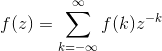Where, F(k) is a discrete data
Z is a complex number
F (z) is Fourier transform of f (k).

Important Properties of z transformation are written below
Linearity
Let us consider summation of two discrete functions f (k) and g (k) such that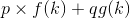such that p and q are constants, now on taking the Laplace transform we have by property of linearity:Change of Scale: let us consider a function f(k), on taking the z transform we have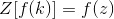then we have by change of scale propertyShifting Property: As per this propertyNow let us discuss some important z transforms and I will suggest readers to learn these transforms: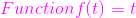Laplace transformation of this function is 1/s2 and the corresponding f(k) = kT. Now the z transformation of this function is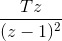Function f (t) = t2: Laplace transformation of this function is 2/s3 and the corresponding f(k) = kT. Now the z transformation of this function is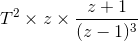Laplace transformation of this function is 1/(s + a) and the corresponding f(k) = e(-akT). Now the z transformation of this function is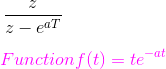Laplace transformation of this function is 1/(s + a)2 and the corresponding f(k) = Te-akT. Now the z transformation of this function is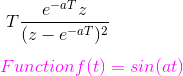Laplace transformation of this function is a/(s2 + a2) and the corresponding f(k) = sin(akT). Now the z transformation of this function isLaplace transformation of this function is s/(s2 + a2) and the corresponding f(k) = cos(akT). Now the z transformation of this function isNow sometime there is a need to sample data again, which means converting discrete data into continuous form. We can convert digital data of control system into continuous form by hold circuits which are discussed below:

Hold Circuits: These are the circuits which converts discrete data into continuous data or original data. Now there are two types of Hold circuits and they are explained in detail:

Zero Order Hold Circuit
The block diagram representation of the zero order hold circuit is given below:
Figure related to zero order hold.
In the block diagram we have given an input f(t) to the circuit, when we allow input signal to pass through this circuit it reconverts the input signal into continuous one. The output of the zero order hold circuit is shown below.
Now we are interested in finding out the transfer function of the zero order hold circuit. On writing the output equation we haveon taking the Laplace transform of the above equation we have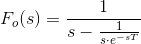From the above equation we can calculate transfer function as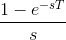On substituting s=jω we can draw the bode plot for the zero order hold circuit. The electrical representation of the zero order hold circuit is shown below, which consists of a sampler connected in series with a resistor and this combination is connected with a parallel combination of resistor and capacitor.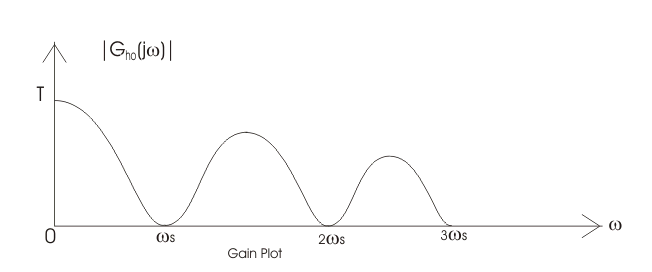GAIN PLOT – frequency response curve of ZOH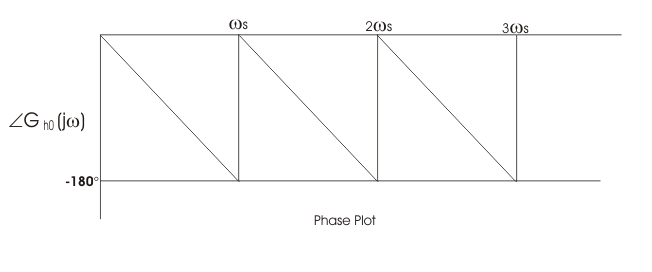PHASE PLOT – frequency response curve of ZOH

First Order Hold Circuit
The block diagram representation of the first order hold circuit is given below:First Order Hold Circuit

In the block diagram we have given an input f(t) to the circuit, when we allow input signal to pass through this circuit it reconverts the input signal into continuous one. The output of the first order hold circuit is shown below: Now we are interested in finding out the transfer function of the first order hold circuit. On writing the output equation we haveOn taking the Laplace transform of the above equation we have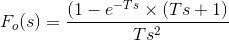From the above equation we can calculate transfer function as (1-e-sT)/s. on substituting s=jω we can draw the bode plot for the zero order hold circuit. The bode plot for the first order hold circuit is shown below which consists of a magnitude plot and a phase angle plot.The magnitude plot starts with magnitude value 2π/ωs.Gain Plot of First Order Hold Circuit

Want To Learn Faster? 🎓
Get electrical articles delivered to your inbox every week.
No credit card required—it’s 100% free.